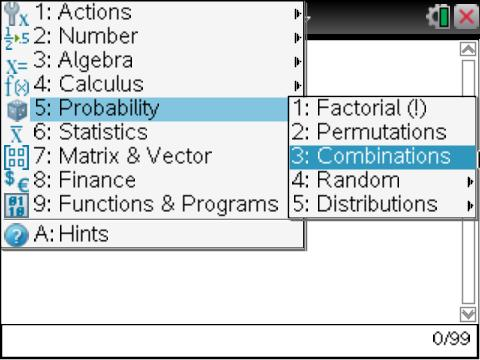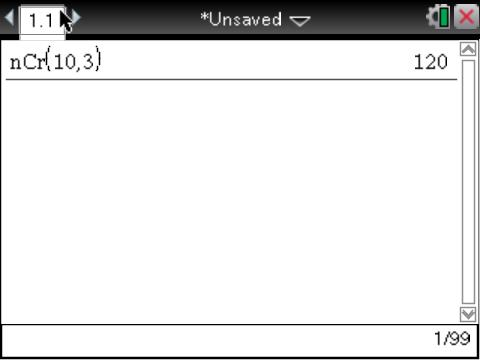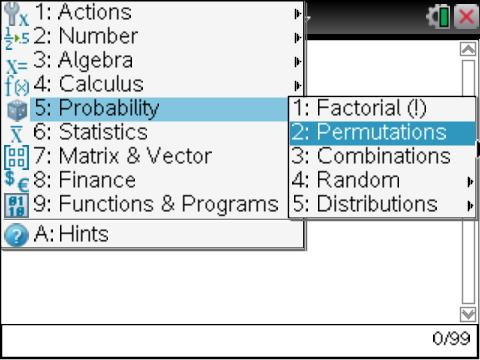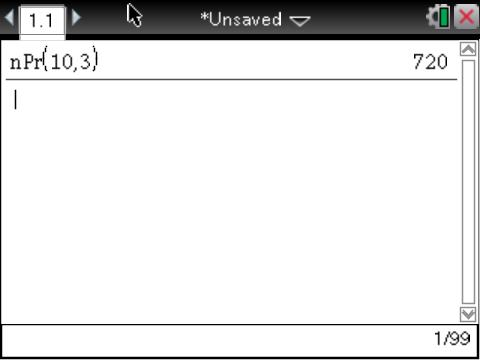# Knowledge Base

## Solution 29710: Calculating Combinations and Permutations on the TI-Nspire™ Family Products.

### How do I calculate combinations and permutations on the TI-Nspire family products?

The examples below will demonstrate how to calculate combinations and permutations using the TI-Nspire family handhelds and computer software.

Example:

How many possible combinations of picking a team of 3 people out of a group of 10 people?

This example would be calculated using combinations, since the order of the people being chosen doesn't matter. The equation is nCr = (n!)/((n-k)!k!), where n is the number of participants, and k is the number of people we are choosing.

On the calculator screen:

1) Press [Menu]   to select nCr(
2) Input 10,3)
3) Press [Enter]Example:

How many possible permutations of first, second, and third place be awarded in a race between 10 people?

This example would be calculated using permutations, since the order of the results matter. The equation is nPr = (n!)/(n-k)!, where n is the number of participants, and k is the number of people that receive medals.

On the calculator screen:

1) Press [Menu]   to select nPr(
2) Input 10,3)
3) Press [Enter]